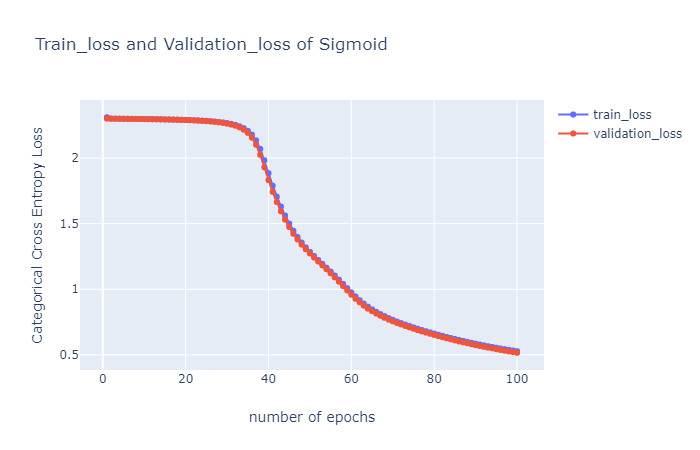# Activation Functions : Why “tanh” outperforms “logistic sigmoid”.

Source: Deep Learning on Mediumtanh function is symmetric about the origin, where the inputs would be normalized and they are more likely to produce outputs (which are inputs to next layer), and they are on an average close to zero.

It can also be said that data is centered around zero for tanh (centered around zero is nothing but mean of the input data is around zero.

These are the main reasons why tanh is preferred and performs better than sigmoid (logistic).

## Why you should normalize ?

Assume, all the inputs are positive. Weights to a particular node in the first weight layer are updated by an amount proportional to δx where δ is the (scalar) error at that node and x is the input vector. When all of the components of an input vector are positive, all updates of weights that feed into a node will have the same sign (i.e. sign of (δ)). As a result, these weights can only all decrease or all increase together for a given input pattern. Thus, if a weight vector must change direction it can only do so by zigzagging which is inefficient and thus very slow. — — To prevent such cases, normalization should be done where the average becomes zero.

This approach(normalization) should be applied at all the layers in the network, which means that we want the average of the outputs of a node to be close to zero because these outputs are the inputs to the next layer.

Convergence is usually faster if the average of each input variable over the training set is close to zero.

The network training converges faster if its inputs are whitened — i.e., linearly transformed to have zero means and unit variances and decorrelated. As each layer observes the inputs produced by the layers below, it would be advantageous to achieve the same whitening of the inputs of each layer.

## Getting stuck during training (train time):

1. Logistic sigmoid can cause a neural network to get “stuck” during training. This is because, if a strongly-negative input is provided to the logistic sigmoid, it outputs values, which are very near to zero. Due to the fact that, neural networks use the feed-forward activations to calculate parameter gradients and these gradients will be less regularly updated than it should be because of this behaviour of logistic sigmoid. In simple terms, weights which are updated through backpropagation will be quite slow.
2. In contrast to it, In tanh as the output values range between (1,-1). So, strongly negative inputs to the tanh will map to negative outputs. Additionally, only zero-valued inputs are mapped to near-zero outputs. These properties make the network less likely to get “stuck” during training.

## Problem of tanh and advantages of logistic sigmoid:

1. tanh functions — error surface can be very flat at origin. So,initializing very small weights should be avoided.
2. Error surfaces can also be flat far from origin because of saturation of sigmoids (saturation is nothing but, the values can’t be beyond the limits, For instance, the value of logistic sigmoid can’t be above 1 or below 0).

These both are problems of tanh and sigmoids.

1. Logistic Sigmoid has beautiful probabilistic interpretation, which made it more popular. Rather than classifying 0 or 1, logistic sigmoid can give the probability value of particular data point belonging to 0 or 1.
2. Such interpretation lacks with tanh function.

This is the main advantage of logistic sigmoid.